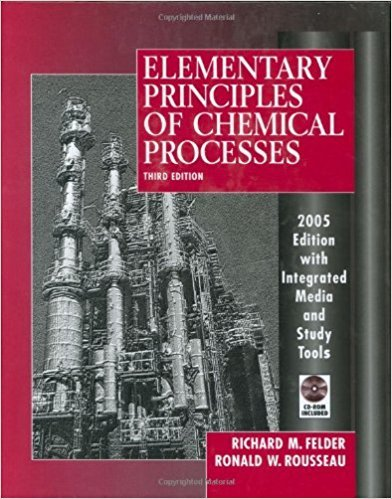×
Get Full Access to Elementary Principles Of Chemical Processes - 3 Edition - Chapter 6 - Problem 6.7
Get Full Access to Elementary Principles Of Chemical Processes - 3 Edition - Chapter 6 - Problem 6.7

×

# Estimate the vapor pressure of acetone (mm Hg) at 50C (a)ISBN: 9780471687573 143

## Solution for problem 6.7 Chapter 6

Elementary Principles of Chemical Processes | 3rd Edition

• Textbook Solutions
• 2901 Step-by-step solutions solved by professors and subject experts
• Get 24/7 help from StudySoup virtual teaching assistantsElementary Principles of Chemical Processes | 3rd Edition

4 5 1 431 Reviews
22
3
Problem 6.7

Estimate the vapor pressure of acetone (mm Hg) at 50C (a) from data in Perry's Chemical Engineers' Handbook and the Clausius-Clapeyron equation, (b) from the Cox chart (Figure 6.1-4), and (c) from the Antoine equation using parameters from Table BA.

Step-by-Step Solution:

Problem 6.7

Estimate the vapor pressure of acetone (mm Hg) at 50C (a) from data in Perry's Chemical Engineers' Handbook and the Clausius-Clapeyron equation, (b) from the Cox chart (Figure 6.1-4), and (c) from the Antoine equation using parameters from Table BA.

Step by step solution

Step 1 of 3

a)

From data in Perry's Chemical Engineers' Handbook,

The value ofis not provided.

If the data fits the Clausius -Clapeyron equation, then the natural logarithmic of the partial pressure and Temperature in Kelvinswill form a linear relationship with a slope, “a” and intercept “b”.

The slope and intercept are then:

Let’s calculate the “a” and “b” values.By using these slope and intercept values, we can calculate the partial pressure for acetone atusing this method..

Therefore, estimated vapour pressure of acetone atis 569.58 mm Hg.

_________________________________________________________________________

Step 2 of 3

Step 3 of 3

##### ISBN: 9780471687573

Unlock Textbook Solution